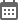# MAH CET MBA Rank Predictor 2021

1. Input your estimated MAH CET Exam 2021 score

2. Get your predicted Rank based on your estimated MAH CET 2021 score## MAH CET Rank Predictor 2021

MAH CET Rank Predictor 2021: Careers360 presents MAH CET 2021 rank predictor for students who want to know their rank in MAH CET 2021. With the help of MAH CET percentile predictor 2021, candidates can know their probable rank and percentile even before the official announcement of MAH CET result 2021. The rank predictor of MAH CET is the best tool to know MAH CET MBA score vs rank.This MAH CET rank predictor can also help you draw an idea about

The Maharashtra Common Entrance Test (MAH CET) is a state-level entrance examination that holds great importance for Management aspirants. A good rank in MAH CET exam 2021 will be the deciding factor for MMS/MBA admission to JBIMSPUMBAKJ SIMSRSIMSREE, and other top colleges accepting MAH CET score etc. Read further to know how to and why to use MAH CET rank predictor.

How to use MAH CET MBA Rank Predictor 2021?

Given below are the steps for using the rank predictor for MAH CET MBA 2021:

• Enter your expected score out of 200.
• Choose the category and disability of candidates.
• Next, enter your MAH CET 2021 registration number (Optional).
• At last, students have to enter their date of birth.
• Click on the ‘Predict My Rank’ button.
• The MAH CET MBA 2021 rank predictor results shall appear on the screen.

MAH CET Rank Predictor 2021: How to calculate score?

The MAH  CET scores are calculated using the marking scheme. One mark will be awarded for every right answer and there will be no negative mark for any incorrect answer, as per the marking scheme of MAH CET 2021. To calculate the MAH CET exam scores, the total number of correct answers is multiplied by 1. Candidates can understand the process of calculating the MAH CET 2021 scores by checking the example provided below.

For example: Suppose a student has answered 60 questions out of 200 correctly then 60 will be multiplied by 1. Hence, the final score of the student will be 60.

MAH CET score vs percentile

The MAH CET result 2021 will be released 1 to 2 weeks after the exam day. The scorecard of MAH MBA CET will mention the scores and percentile of the student scores in the examination. The table below will help you to know the expected percentile of MAH MBA CET for different score ranges.

MBA CET marks vs percentile

 MAH CET MBA Score MAH CET MBA Percentile 160+ 99.50+ 140-160 99+ 120-140 98+ 100-120 95+ 90-100 90+

MAH CET Percentile Score = 100 * (Number of students in the exam with normalized marks ≤ the student) / Total number of students in the exam.

Your probable MAH CET rank can be:

• Probable Rank = (100 - percentile score) X (number of candidates appeared for the entrance test)/ 100

Example:

Let us assume that 200 students appeared for the MAH CET MBA exam. The percentile of two candidates who have appeared for the exam might be as follows:

 Students Name All India Rank (AIR) MAH CET Percentile ABC 2 Percentile = 200 - 2/200 x 100 = 99 XYZ 40 Percentile = 200 - 40/200 x 100 = 80

MAH MBA CET 2021 - Marking Scheme

Based on the marking scheme of MAH MBA CET 2021,

• Students will be awarded +1 mark for each correct answer.
• For incorrect answers/ unanswered questions, there will be no negative marking.

MAH CET MBA 2021 Rank Predictor - Key features

• The MAH MBA CET percentile predictor lets students predict their percentile before the results are announced.
• The accuracy of MAH CET MBA 2021 rank predictor from percentile score depends upon the details entered by the students.
• MAH CET MBA rank predictor 2021 indicates the percentile for the difficulty level of the exam shift based on student’s experience and expert analysis of MAH CET exam.

MAH CET Result 2021

State Common Entrance Test Cell, Maharashtra will announce the MAH CET MBA results in online mode on its official website. Candidates can check their MAH CET result using their login credentials.

The MAH CET result will include All India Rank (AIR) and the scorecard where the total percentile and score will be mentioned.

### Other Rank Predictors